# Voltage Follower

## Chapter 5 - Discrete Semiconductor Circuits

PARTS AND MATERIALS

• One NPN transistor—models 2N2222 or 2N3403 recommended (Radio Shack catalog # 276-1617 is a package of fifteen NPN transistors ideal for this and other experiments)
• Two 6-volt batteries
• Two 1 kΩ resistors
• One 10 kΩ potentiometer, single-turn, linear taper (Radio Shack catalog # 271-1715)

Beware that not all transistors share the same terminal designations, or pinouts, even if they share the same physical appearance. This will dictate how you connect the transistors together and to other components, so be sure to check the manufacturer’s specifications (component datasheet), easily obtained from the manufacturer’s website.

Beware that it is possible for the transistor’s package and even the manufacturer’s datasheet to show incorrect terminal identification diagrams! Double-checking pin identities with your multimeter’s “diode check” function is highly recommended.

For details on how to identify bipolar transistor terminals using a multimeter, consult chapter 4 of the Semiconductor volume (volume III) of this book series.

CROSS-REFERENCES

Lessons In Electric Circuits, Volume 3, chapter 4: “Bipolar Junction Transistors

LEARNING OBJECTIVES

• Purpose of circuit “ground” when there is no actual connection to earth ground
• Using a shunt resistor to measure current with a voltmeter
• Measure amplifier voltage gain
• Measure amplifier current gain
• Amplifier impedance transformation

SCHEMATIC DIAGRAM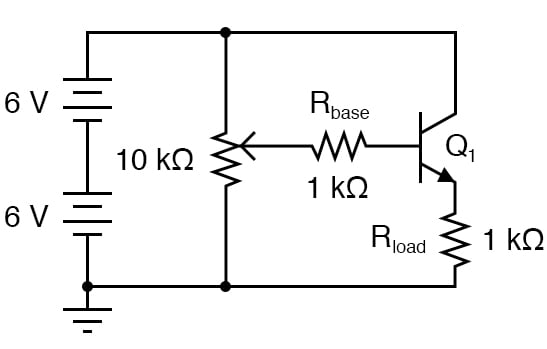ILLUSTRATION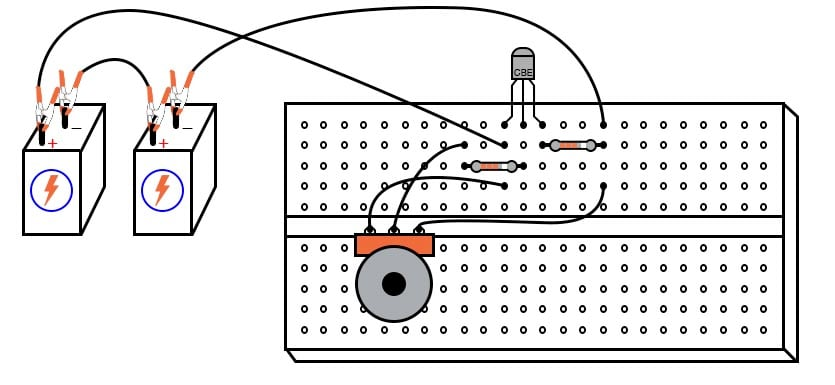INSTRUCTIONS

Again, beware that the transistor you select for this experiment may not have the same terminal designations shown here, and so the breadboard layout shown in the illustration may not be correct for you. In my illustrations, I show all TO-92 package transistors with terminals labeled “CBE”: Collector, Base, and Emitter, from left to right.

This is correct for the model 2N2222 transistor and some others, but not for all; not even for all NPN-type transistors! As usual, check with the manufacturer for details on the particular component(s) you choose for a project.

With bipolar junction transistors, it is easy enough to verify terminal assignments with a multimeter. The voltage follower is the safest and easiest transistor amplifier circuit to build.

Its purpose is to provide approximately the same voltage to a load as what is input to the amplifier but at a much greater current. In other words, it has no voltage gain, but it does have current gain.

Note that the negative (-) side of the power supply is shown in the schematic diagram to be connected to ground, as indicated by the symbol in the lower-left corner of the diagram. This does not necessarily represent a connection to the actual earth.

What it means is that this point in the circuit—and all points electrically common to it—constitute the default reference point for all voltage measurements in the circuit. Since voltage is by necessity a quantity relative between two points, a “common” point of reference designated in a circuit gives us the ability to speak meaningfully of voltage at particular, single points in that circuit.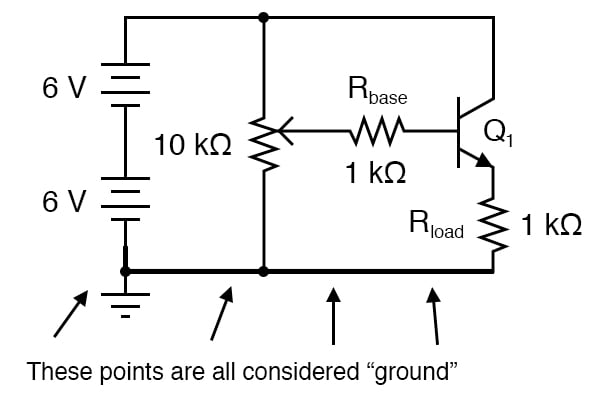For example, if I were to speak of voltage at the base of the transistor (VB), I would mean the voltage measured between the transistor’s base terminal and the negative side of the power supply (ground), with the red probe touching the base terminal and the black probe touching ground. Normally, it is nonsense to speak of voltage at a single point, but having an implicit reference point for voltage measurements makes such statements meaningful: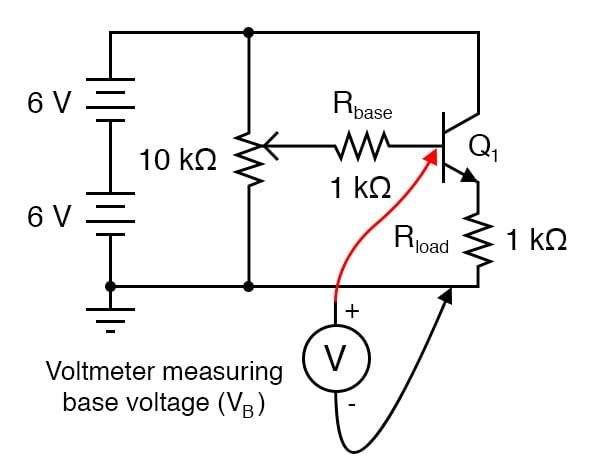Build this circuit, and measure output voltage versus input voltage for several different potentiometer settings. Input voltage is the voltage at the potentiometer’s wiper (voltage between the wiper and circuit ground), while output voltage is the load resistor voltage (voltage across the load resistor, or emitter voltage: between the emitter and circuit ground).

You should see a close correlation between these two voltages: one is just a little bit greater than the other (about 0.6 volts or so?), but a change in the input voltage gives almost equal change in the output voltage. Because the relationship between input change and output change is almost 1:1, we say that the AC voltage gain of this amplifier is nearly 1.

Not very impressive, is it? Now measure current through the base of the transistor (input current) versus current through the load resistor (output current). Before you break the circuit and insert your ammeter to take these measurements, consider an alternative method: measure voltage across the base and load resistors, whose resistance values are known.

Using Ohm’s Law, current through each resistor may be easily calculated: divide the measured voltage by the known resistance (I=E/R). This calculation is particularly easy with resistors of 1 kΩ value: there will be 1 milliamp of current for every volt of drop across them.

For best precision, you may measure the resistance of each resistor rather than assume an exact value of 1 kΩ, but it really doesn’t matter much for the purposes of this experiment. When resistors are used to take current measurements by “translating” a current into a corresponding voltage, they are often referred to as shunt resistors.

You should expect to find huge differences between input and output currents for this amplifier circuit. In fact, it is not uncommon to experience current gains well in excess of 200 for a small-signal transistor operating at low current levels.

This is the primary purpose of a voltage follower circuit: to boost the current capacity of a “weak” signal without altering its voltage. Another way of thinking of this circuit’s function is in terms of impedance.

The input side of this amplifier accepts a voltage signal without drawing much current. The output side of this amplifier delivers the same voltage, but at a current limited only by load resistance and the current-handling ability of the transistor.

Cast in terms of impedance, we could say that this amplifier has a high input impedance (voltage dropped with very little current drawn) and a low output impedance (voltage dropped with almost unlimited current-sourcing capacity).

COMPUTER SIMULATION

Schematic with SPICE node numbers: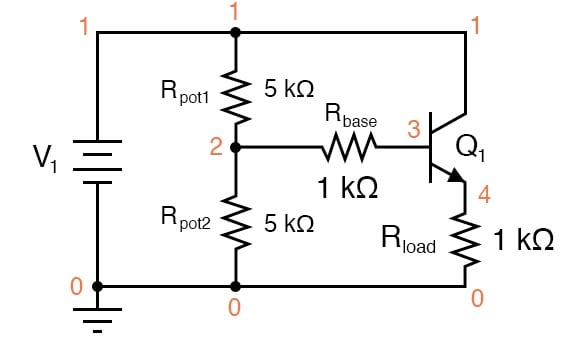Netlist (make a text file containing the following text, verbatim):

Voltage follower v1 1 0 rpot1 1 2 5k rpot2 2 0 5k rbase 2 3 1k rload 4 0 1k q1 1 3 4 mod1 .model mod1 npn bf=200 .dc v1 12 12 1 .print dc v(2,0) v(4,0) v(2,3) .end

When this simulation is run through the SPICE program, it shows an input voltage of 5.937 volts and an output voltage of 5.095 volts, with an input current of 25.35 µA (2.535E-02 volts dropped across the 1 kΩ Rbase resistor). Output current is, of course, 5.095 mA, inferred from the output voltage of 5.095 volts dropped across a load resistance of exactly 1 kΩ.

You may change the “potentiometer” setting in this circuit by adjusting the values of Rpot1 and Rpot2, always keeping their sum at 10 kΩ.

RELATED WORKSHEET: